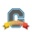cancel
Showing results for
Show  only  | Search instead for
Did you mean:Community Contributor

## Weight all quizzes equally within a weighted category

So, this is a new one for me.  I have several faculty who want an Assignment group for Quizzes that is weighted (they want to apply some rules).  Within that group that have about 45 quizzes.  The quizzes all have different # of questions, BUT they want them all to be weighted equally within the group.  SO, if a student is absent on a day that the quiz has 10 questions will not be penalized more than a student who misses a day when the quiz only has 2 questions.

Does that make sense?  Does anyone have a suggestion?

Thanks,

Heidi

1 SolutionCommunity Coach

@heidi1 ​, the only way to equally weight quizzes within a weighted Assignment group is to make all of the quizzes worth the same number of points. The number of points doesn't really matter, but it has to be the same.

Ex: All quizzes are worth 10 points and then divide by the number of questions to get how many points each question needs to be worth. If a quiz has 10 questions then each question is worth 1 point (10 points/10 questions). If a quiz has 2 questions, then each question is worth 5 points (10 points/ 2 questions). If a quiz has 7 questions, then each question is worth (approximately) 1.42857 (10 points/ 7 questions).

Could this get ugly? Yes, it probably will (example with the 7 question quiz above), and there will probably be some issues with rounding. Yet, if there are a different numbers of questions for each quiz this is the only solution that will keep all quizzes worth the same weight within the Assignment group.

Hope this helps!

6 RepliesCommunity Coach

@heidi1 ​, the only way to equally weight quizzes within a weighted Assignment group is to make all of the quizzes worth the same number of points. The number of points doesn't really matter, but it has to be the same.

Ex: All quizzes are worth 10 points and then divide by the number of questions to get how many points each question needs to be worth. If a quiz has 10 questions then each question is worth 1 point (10 points/10 questions). If a quiz has 2 questions, then each question is worth 5 points (10 points/ 2 questions). If a quiz has 7 questions, then each question is worth (approximately) 1.42857 (10 points/ 7 questions).

Could this get ugly? Yes, it probably will (example with the 7 question quiz above), and there will probably be some issues with rounding. Yet, if there are a different numbers of questions for each quiz this is the only solution that will keep all quizzes worth the same weight within the Assignment group.

Hope this helps!Community Coach

PS - Here's a recently archived feature idea that sounds like what you are wanting -Community Contributor

Thanks Kona, you're the best!

HeidiCommunity Coach

Happy to help!Community Member

I just discovered this at the end of a course. I have 4 quizes and drop the lowest one, but they have different point values. But, per the syllabus, each quiz is worth 20% of the course grade, or 33% of the Quiz category. But, because the point values are different on the quizzes, Canvas is weighting the higher-point quizzes more than the lower point ones. Is there a way to change this after the fact such that each quiz is worth the same amount?

Thanks,
Matt RobertsCommunity Member

My thought is that I would need to go in and create new quizzes that correspond to each quiz with equal point values and then transfer the percentages from the orginal quizzes. But, if there an easier way?

Thanks,

Matt Roberts